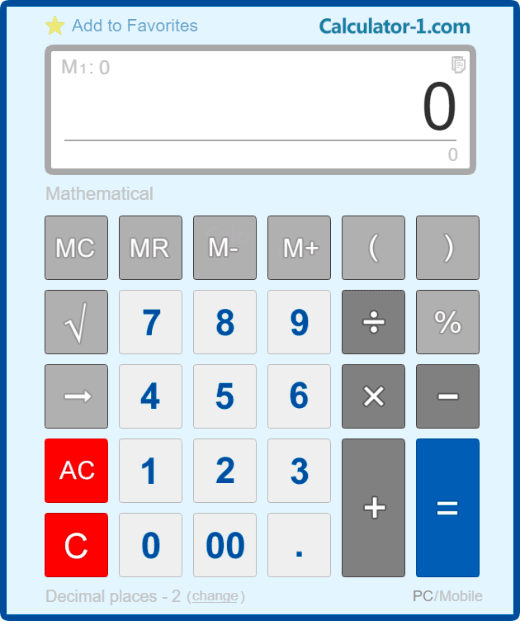# Online Calculator### Large, easy and convenient online calculator

Use for work, school or personal calculations. You can make not only simple math calculations and calculation of interest on the loan and bank lending rates, the calculation of the cost of works and utilities.

Commands for the online calculator you can enter not only the mouse, but with a digital computer keyboard. Detailed instructions for using the calculator, see below.

## Instructions for using the online calculator

### Keys function

[ 0 ], [ 1 ], [ 2 ], ... [ 9 ] - standard number keys;
[ 00 ] - key input 2 zeros;
[ → ] - remove the last character on the display;
[ +/- ] - change the mathematical sign of;
[ XY ] - calculation of X to the power of Y;
[ √ ] - calculate the square root;
[ + ] - addition, [ - ] - subtraction, [ х ] - multiplication, [ ÷ ] - division;
[ % ] - calculates percentages;
[ M+ ] - stored in the memory with the sign [ + ];
[ M- ] - stored in the memory with the sign [ - ];
[ MR ] - get the contents of memory;
[ MC ] - erases the memory content;
[ AC ] - reset the calculator and reset the memory;
[ C ] - resets the calculator without resetting the memory.

### Examples of calculations on the online calculator

Calculate the square root of 529: 529 [ √ ]. The result is equal to 23.
Raise the number 3 to a power 4: 3 [ XY ] 4 [ = ]. The result is equal to 81.
Calculation of percentage of the number of: 500 [ х ] 25 [ % ]. The result is equal to 125.
Calculating what percent is one number of another number: 25 [ ÷ ] 500 [ % ]. The result is equal to 5(%).
Adding percentage to the number: 500 [ + ] 25 [ % ]. The result is equal to 625.
Deduction percentage of the number: 500 [ - ] 25 [ % ]. The result is equal to 375.

### Enter commands from the keyboard PC/Mac

To use free online calculator you can use both ordinary numeric buttons at the top of a keyboard and numeric buttons on the right of a keyboard.

To enter [ = ] - key [Enter].
To erase the last character - [Backspace] (arrow keys).
To enter [ + ] - key [ + ] at the top or [ + ] key on the numeric keypad on the right.
To enter [ - ] - key [ - ] at the top or [ - ] key on the right.
To enter [ x ] (multiplication) - key [ * ] on the numeric keypad on the right or a combination of keys[ * ] and [ Shift ].
To enter [ ÷ ] (divide) - key [ / ] on the numeric keypad on the right or a combination of keys [ : ] and [ Shift ].